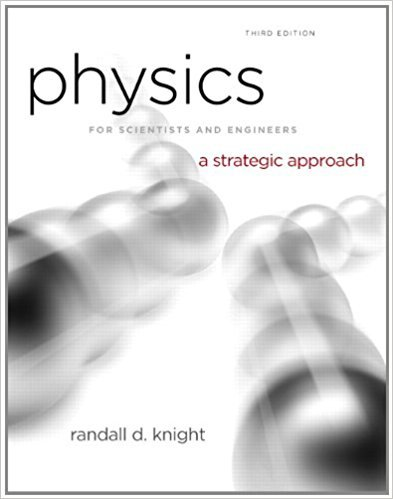×
Get Full Access to Physics For Scientists And Engineers: A Strategic Approach With Modern Physics - 3 Edition - Chapter 14 - Problem 9cq
Get Full Access to Physics For Scientists And Engineers: A Strategic Approach With Modern Physics - 3 Edition - Chapter 14 - Problem 9cq

×

# FIGURE shows the potential-energy diagram and the totalISBN: 9780321740908 69

## Solution for problem 9CQ Chapter 14

Physics for Scientists and Engineers: A Strategic Approach with Modern Physics | 3rd Edition

• Textbook Solutions
• 2901 Step-by-step solutions solved by professors and subject experts
• Get 24/7 help from StudySoup virtual teaching assistantsPhysics for Scientists and Engineers: A Strategic Approach with Modern Physics | 3rd Edition

4 5 1 433 Reviews
14
0
Problem 9CQ

Problem 9CQ

FIGURE shows the potential-energy diagram and the total energy line of a particle oscillating on a spring.

a. What is the spring’s equilibrium length?

b. Where are the turning points of the motion? Explain.

c. What is the particle’s maximum kinetic energy?

d. What will be the turning points if the particle’s total energy is doubled?

FIGUREStep-by-Step Solution:

Solution 9CQ

Step 1 of 5

For a given figure, potential energy diagram and total energy line, for a particle oscillating on a spring, we need to find the following;

Step 2 of 5

Step 3 of 5

##### ISBN: 9780321740908

The answer to “FIGURE shows the potential-energy diagram and the total energy line of a particle oscillating on a spring.a. What is the spring’s equilibrium length?________________b. Where are the turning points of the motion? Explain.________________c. What is the particle’s maximum kinetic energy?________________d. What will be the turning points if the particle’s total energy is doubled?FIGURE” is broken down into a number of easy to follow steps, and 52 words. This full solution covers the following key subjects: Energy, particle, points, spring, turning. This expansive textbook survival guide covers 17 chapters, and 1439 solutions. Since the solution to 9CQ from 14 chapter was answered, more than 2456 students have viewed the full step-by-step answer. The full step-by-step solution to problem: 9CQ from chapter: 14 was answered by , our top Physics solution expert on 08/30/17, 04:34AM. This textbook survival guide was created for the textbook: Physics for Scientists and Engineers: A Strategic Approach with Modern Physics, edition: 3. Physics for Scientists and Engineers: A Strategic Approach with Modern Physics was written by and is associated to the ISBN: 9780321740908.

Unlock Textbook Solution